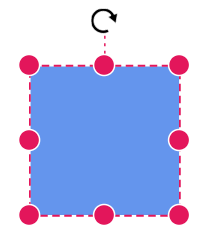# Node Interaction in Blazor Diagram Component

25 Aug 20239 minutes to read

Diagram provides the support to select, drag, resize, or rotate the node interactively.

## How to select the node

A node can be selected at runtime by using the Select method and clear the selection in the diagram by using the ClearSelection. The following code explains how to select and clear selection in the diagram.

``````@using Syncfusion.Blazor.Diagram
@using System.Collections.ObjectModel
@using Syncfusion.Blazor.Buttons

<SfButton Content="Select" OnClick="@OnSelect" />
<SfButton Content="UnSelect" OnClick="@UnSelect" />
<SfDiagramComponent @ref="@diagram" Height="600px" Nodes="@nodes" />

@code
{
// Reference of the diagram
SfDiagramComponent diagram;
// To define node collection
DiagramObjectCollection<Node> nodes;

protected override void OnInitialized()
{
nodes = new DiagramObjectCollection<Node>();
// A node is created and stored in nodes collection.
Node node = new Node()
{
// Position of the node
OffsetX = 250,
OffsetY = 250,
// Size of the node
Width = 100,
Height = 100,
Style = new ShapeStyle() { Fill = "#6495ED", StrokeColor = "white" }
};
}

public void OnSelect()
{
// Select the node
diagram.Select(new ObservableCollection<IDiagramObject> { diagram.Nodes });
}

public void UnSelect()
{
// clear selection in the diagram
diagram.ClearSelection();
}
}``````

And also the selection enable during the interaction.

• An element can be selected by clicking that element.
• When you select the elements in the diagram, the SelectionChanging and SelectionChanged events get triggered and do customization on those events.## How to drag the node

A node can be dragged at runtime by using the Drag method. The following code explains how to drag the node by using the drag method.

``````@using Syncfusion.Blazor.Diagram
@using Syncfusion.Blazor.Buttons

<SfButton Content="Drag" OnClick="@OnDrag" />
<SfDiagramComponent @ref="@Diagram" Height="600px" Nodes="@nodes" />

@code
{
// Reference of the diagram
SfDiagramComponent Diagram;
// To define node collection
DiagramObjectCollection<Node> nodes;

protected override void OnInitialized()
{
nodes = new DiagramObjectCollection<Node>();
// A node is created and stored in nodes collection.
Node node = new Node()
{
// Position of the node
OffsetX = 250,
OffsetY = 250,
// Size of the node
Width = 100,
Height = 100,
Style = new ShapeStyle() { Fill = "#6495ED", StrokeColor = "white" }
};
}

public void OnDrag()
{
// Drag the node
Diagram.Drag(Diagram.Nodes, 10, 10);
}
}``````

Also, drag the node during the interaction.

• An object can be dragged by clicking and dragging it. When multiple elements are selected, dragging any one of the selected elements move all the selected elements.
• When you drag the elements in the diagram, the PositionChanging and PositionChanged events get triggered and do customization on those events.## How to resize the node

A node can be resized at runtime by using the Scale method. The following code explains how to resize the node by using the scale method.

``````@using Syncfusion.Blazor.Diagram
@using Syncfusion.Blazor.Buttons

<SfButton Content="Resize" OnClick="@OnResize" />
<SfDiagramComponent @ref="@diagram" Height="600px" Nodes="@nodes" />

@code
{
// Reference of the diagram
SfDiagramComponent diagram;
// To define node collection
DiagramObjectCollection<Node> nodes;

protected override void OnInitialized()
{
nodes = new DiagramObjectCollection<Node>();
// A node is created and stored in nodes collection.
Node node = new Node()
{
// Position of the node
OffsetX = 250,
OffsetY = 250,
// Size of the node
Width = 100,
Height = 100,
Style = new ShapeStyle() { Fill = "#6495ED", StrokeColor = "white" }
};
}

public void OnResize()
{
// Resize the node
diagram.Scale(diagram.Nodes, 0.5, 0.5, new DiagramPoint() { X = 0, Y = 0 });
}
}``````

Also, you can resize the node during interaction.

• Selector is surrounded by eight thumbs. When dragging these thumbs, the selected items can be resized.
• When one corner of the selector is dragged, the opposite corner will be in a static position.
• When a node is resized, the SizeChanging and SizeChanged events get triggered.## How to rotate the node

A node can be rotated at runtime by using the Rotate method. The following code explains how to rotate the node by using the rotate method.

``````@using Syncfusion.Blazor.Diagram
@using Syncfusion.Blazor.Buttons

<SfButton Content="Rotate" OnClick="@OnRotate" />
<SfDiagramComponent @ref="@diagram" Height="600px" Nodes="@nodes" />

@code
{
// Reference of the diagram
SfDiagramComponent diagram;
// To define node collection
DiagramObjectCollection<Node> nodes;

protected override void OnInitialized()
{
nodes = new DiagramObjectCollection<Node>();
// A node is created and stored in nodes collection.
Node node = new Node()
{
// Position of the node
OffsetX = 250,
OffsetY = 250,
// Size of the node
Width = 100,
Height = 100,
Style = new ShapeStyle() { Fill = "#6495ED", StrokeColor = "white" }
};
}

public void OnRotate()
{
// Rotate the node
diagram.Rotate(diagram.Nodes, diagram.Nodes.RotationAngle + 10);
}
}``````

Also, rotate the node during the interaction.

• A rotate handler is placed above the selector. Clicking and dragging the handler in a circular direction lead to rotate the node.
• The node is rotated with reference to the static pivot point.
• Pivot thumb (thumb at the middle of the node) appears when rotating the node to represent the static point.
• When a node is rotated, the RotationChanging and RotationChanged events get triggered.## How to flip the node

The Flip is performed to give the mirrored image of the original element.